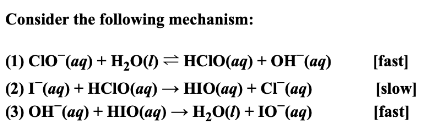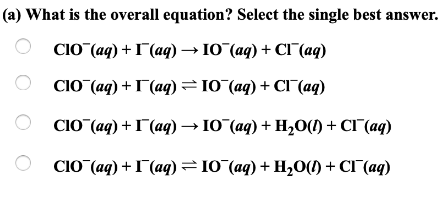# Consider the following mecahnism: (1) ClO^-(aq) + H2O(l) ⇌ HClO(aq) + OH^-(aq) [fast] (2) I^-(aq) + HClO(aq) → HIO(aq) + Cl^-(aq) [slow] (3) OH^-(aq) + HIO(aq) → H2O(l) + IO^-(aq) [fast] (a) What is the overall equation? Select the single best answer.